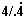# Funny Arithmetic

 =
 Base digit 1 2 3 4 5 6 7 8 9 Number of occurrences 1 2 3 4 5 6 7 8 9 10 11 12 13 14 15 16 17 18 19 20 21 Goal

Using arithmetic, logical, and bitwise shift operators and parenthesis, build an arithmetic expression with as many Base digits as specified in the Number of occurrences. Your expression should evaluate to the specified Goal. For example, for the Base digit 3 and Number of occurences 5 the following goals can be met
• 1 = 3/3 + (3-3)/3
• 2 = (3&3)/3 + 3/3
• ...
• 6 = 3<<3/3·3/3
• ...
• 10 = 33/3 - 3/3
• etc.

The following operators are allowed:

• arithmetic
• -, subtraction
• ·, multiplication
• /, division
• %, modulus
• logical (bitwise)
• &, conjunction  & 0 1 0 0 0 1 0 1
• |, disjunction  | 0 1 0 0 1 1 1 1
• ^, exclusive or  ^ 0 1 0 0 1 1 1 0
• bitwise shift
• <<, left shift. For example,
3<<2=12, 5<<1=10
• >>, right shift. For example,
5>>1=2, 13>>2=3

You can use parenthesis () and braces {} but not brackets [] to separate subexpressions.From time to time I am asked questions of number representations related to the above. I'll be orginizing results in tables to keep them easily available. Normally, in this kind of problems, allowed operations are different. I was limited by the JavaScript built-in parsing facility. Thus, in the following pages I shall use operations listed below:

• !(factorial, 5!=5·4·3·2·1, 6!=6·5!)
• !!(double factorial, 5!!=5·3·1, 6!!=6·4·2)
• !(subfactorial, !n = n!·(1/2! - 1/3! + ... + (-1)n/n!), e.g, !4 = 24(1/2! - 1/3! + 1/4!) = 9.)
• ab(power: a to the power of b), (square root)
• .(decimal point: 4/.4=10)
• .(periodic fraction:=4/.444...=9. For simplicity sake I'll write, say, 4/.(4) or with an overline, like, 4/.4)
• [] (whole part: [1.7]=1, [3.6]=3)
• any kind of parentheses

Remark: the whole part function is a powerful addition to the common arithmetic operations and factorials. This can be demonstrated by the surprising, but shallow, identity: [π][e] + [e] = [e][π] + [π].

Everyone can take part in filling these tables or creating new ones. I insist on one condition though:

 Tables may have gaps that would reflect on my current knowledge or cummulative time investment into solving these puzzles. However, I am the only one allowed to create gaps. All others either fill gaps or submit contiguous solutions for a series of numbers.Following is a list of simple facts that frequently proves handy solving this kind of puzzles: for every positive number a:

1. a - a = 0
2. a / a = 1
3. (a + a) / a = 2
4. (a + a + a) / a = 3
5. a = a

For a digit n there are additional useful identities:

1. n / .(n) = 9
2. n / .n = 10
3. nn / n = 11

(A Java applet that could help experiment with this kind of problems is also avaialble.)

Note: Inder Jeet Taneja has gathered a fantastic collection of various one digit representations of numbers from 1 to 1000.• A single formula to express all numbers
• Any Integer with Three 2s
• Representation of numbers with a single 4. The rules and the possibilities
• One 4, a story
• Three 3's
• Representation of numbers with three 4's
• Representation of numbers with three 5's
• Representation of numbers with four 3's
• Representation of numbers with four 4's
• Representation of numbers with four 5's
• A problem of representing 4 by four identical digits
• A problem of representing 6 by three identical digits
• A 9's Fan's Clock
• Make an Identity
• Fun with numbers: place plus/minus signs between the digits
• 24 with One Digit
• Representation of 3 with Three Equal Digits
•